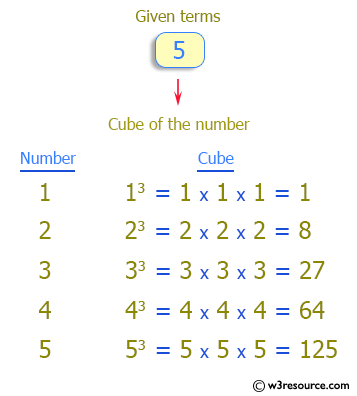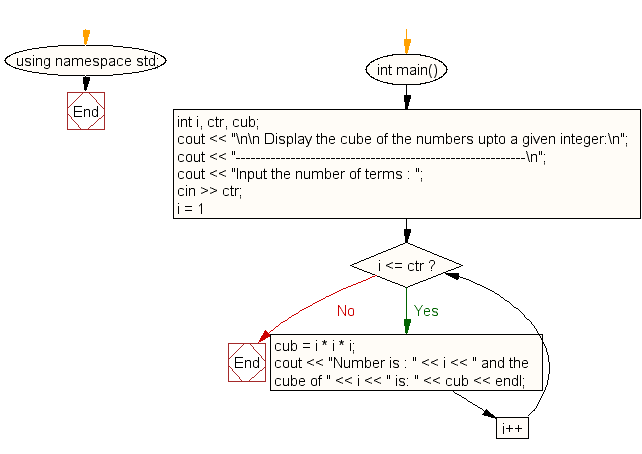﻿ C++ : Display the cube of the number upto given an integer# C++ Exercises: Display the cube of the number upto given an integer

## C++ For Loop: Exercise-18 with Solution

Write a program in C++ to display the cube of the number upto given an integer.

Pictorial Presentation:Sample Solution:-

C++ Code :

``````#include <iostream>
using namespace std;

int main()
{
int i, ctr, cub;

cout << "\n\n Display the cube of the numbers upto a given integer:\n";
cout << "----------------------------------------------------------\n";
cout << "Input the number of terms : ";
cin >> ctr;
for (i = 1; i <= ctr; i++)
{
cub = i * i * i;
cout << "Number is : " << i << " and the cube of " << i << " is: " << cub << endl;
}
}
``````

Sample Output:

``` Display the cube of the numbers upto a given integer:
----------------------------------------------------------
Input the number of terms : 5
Number is : 1 and the cube of 1 is: 1
Number is : 2 and the cube of 2 is: 8
Number is : 3 and the cube of 3 is: 27
Number is : 4 and the cube of 4 is: 64
Number is : 5 and the cube of 5 is: 125
```

Flowchart:C++ Code Editor:

Contribute your code and comments through Disqus.

What is the difficulty level of this exercise?

﻿

## C++ Programming: Tips of the Day

What is a smart pointer and when should I use one?

This answer is rather old, and so describes what was 'good' at the time, which was smart pointers provided by the Boost library. Since C++11, the standard library has provided sufficient smart pointers types, and so you should favour the use of std::unique_ptr, std::shared_ptr and std::weak_ptr.

There was also std::auto_ptr. It was very much like a scoped pointer, except that it also had the "special" dangerous ability to be copied - which also unexpectedly transfers ownership.

It was deprecated in C++11 and removed in C++17, so you shouldn't use it.

```std::auto_ptr<MyObject> p1 (new MyObject());
std::auto_ptr<MyObject> p2 = p1; // Copy and transfer ownership.
// p1 gets set to empty!
p2->DoSomething(); // Works.
p1->DoSomething(); // Oh oh. Hopefully raises some NULL pointer exception.
```

Ref : https://bit.ly/3mc9GHE